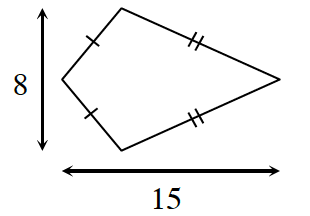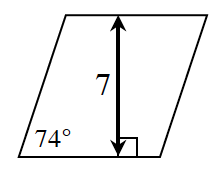### Home > CCG > Chapter 12 > Lesson 12.2.4 > Problem12-101

12-101.

Find the area of each quadrilateral below. Show all work.

1. KiteThe formula for the area of a kite is $\frac{d1 \: \cdot\: d2}{2}$.

$\frac{(8) (15)}{2}$

$\frac{120}{2} =\ 60 \text{ units}^2$

1. RhombusSolve for the length of the base, then find the area.